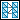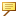To Remove Webs
1. Select a set of triangles.
2. Click Wrap >Remove Webs. The Remove Webs dialog box opens.
3. Type the following values:
Min. Edge Length—Selects triangles based on the length of their edges.
The web triangle edges are longer than those on the actual model. You can select a minimum value for the edge length that is longer than the edge length of the actual model triangles. Adjusting the length selects triangles with a length greater than the specified value.
Min. Angle Deviation—Uses the mathematical normals that extend from the surface to calculate the angle between the normal and the triangles.
The angle values of the model triangles are lower than those of the web triangles. This enables you to specify a minimum angle to distinguish between the model triangles and the web triangles.
To effectively remove webs, adjust both these parameters. The Remove Webs command removes webs that meet at least one of the criteria.
For the removal of webs to be effective, you do not need to select all the triangles, as this operation propagates through the model in a manner similar to the Push Deep command.
4. Click OK.The web removal operation does not consider the distribution of points, and, therefore, cannot distinguish between webs and gaps in the wrap.